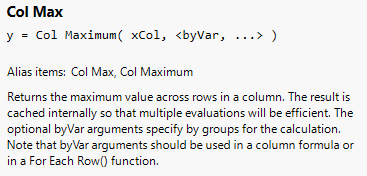Choose Language Hide Translation Bar
Highlighted

## How do I use the Col Maximum Formula with a "where" condition

Hi, I have a problem where I want to formulate a new column as a benchmark to all other countries. If there are more rows for the country, I want to take the max value of it as the benchmark. See the example below for clarification.

Data kind of looks like this:
Country       Value         period

A                  10              201301

A                  30              201301

B                  20              201301

C                  50              201301

D                  40              201301

What I want to achieve (with cell formula): Col Maximum (:value, :period, :country) where :country == "A"

Output:

Country       Value         period        BenchMark

A                  10              201301          30

A                  30              201301          30

B                  20              201301          30

C                  50              201301          30

D                  40              201301          30

As you can see, I want to benchmark the max value of only country A.

I tried Col Maximum (:value, :period, :country == "A") and it will return "50"

Col Maximum (:value, :period, :country) and Col Maximum (:value, :period, :country == "A") both returns "50"

I am pretty sure there's a simple solution for this!

Hope you guys can help!

Thanks thanks!

1 ACCEPTED SOLUTION

Accepted Solutions
Highlighted

## Re: How do I use the Col Maximum Formula with a "where" condition

Given the way you stated your needs, Assuming you know what the country that has the multiple entries.

``````dt = Current Data Table();
rowperiod = :Period[Row()];
Max(
:Value[dt << get rows where(
:Country == "A" & :period == rowperiod
)]
);``````

Jim
11 REPLIES 11
Highlighted

## Re: How do I use the Col Maximum Formula with a "where" condition

Given the way you stated your needs, Assuming you know what the country that has the multiple entries.

``````dt = Current Data Table();
rowperiod = :Period[Row()];
Max(
:Value[dt << get rows where(
:Country == "A" & :period == rowperiod
)]
);``````

Jim
Highlighted

## Re: How do I use the Col Maximum Formula with a "where" condition

Sorry for the late reply! Thanks for the solution!
Highlighted

## Re: How do I use the Col Maximum Formula with a "where" condition

Suppose the country column has several names and I do not know all the possible values. How can this query be tweaked to do that.

I can do that by creating a summary table and then updating the table back, but that seems to be ineffecient method, was curious if it could be done in a single step like this.

Highlighted

## Re: How do I use the Col Maximum Formula with a "where" condition

I typically use the Summarize() function, rather than the Summary Platform, to do easy determination of what levels within a column are found.  Then, from there, you just have to generate the formula's you need to get the job done.

Jim
Highlighted

## Re: How do I use the Col Maximum Formula with a "where" condition

Thanks Jim, I meant summarize only. How I do it is (two jsl commands):

Summarize on the column, create new table with values (min/max/or any other statistic for the column).

Then I update the main table using the summarized table by matching the row entries.

I was just curious if there is conditional option in Max col() command which can identify unique column values and eliminate the need for this extra step. (would eliminate an extra table and an 2 extra steps).

Highlighted

## Re: How do I use the Col Maximum Formula with a "where" condition

See Help > Scripting Index > Functions:Learn it once, use it forever!
Highlighted

## Re: How do I use the Col Maximum Formula with a "where" condition

Hi Mark,
Can you give an example on how to use the <byVar> when using col Max?

thanks.
Highlighted

## Re: How do I use the Col Maximum Formula with a "where" condition

I could add this formula to a new column in the Big Class example data table:

``Col Max( :weight, :age )``
Learn it once, use it forever!
Highlighted

## Re: How do I use the Col Maximum Formula with a "where" condition

Hi Mark,
tried to get maximum weight by age or sex, but only produce one value in the following script.
by ages, there were 6 different ages, for each of them, I'd like to compute the maximum weight for each age.

``````Names Default To Here( 1 );
Clear Log();
dt = Open( "\$SAMPLE_DATA/Big Class.jmp" );
max_weight_by_age = Col Max( :weight, :age );
max_weight_by_sex = Col Max( :weight, :sex );``````
Article Labels

There are no labels assigned to this post.